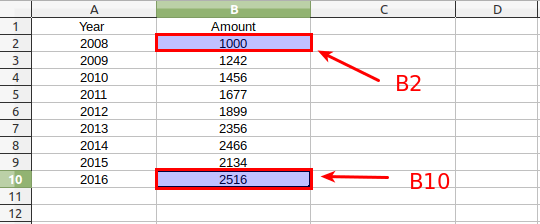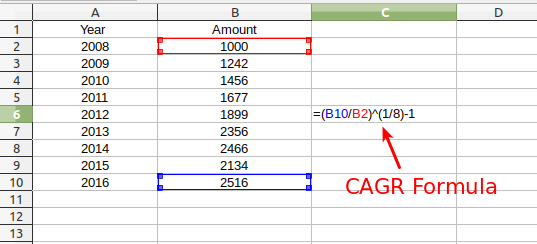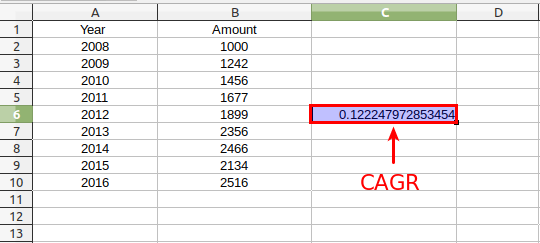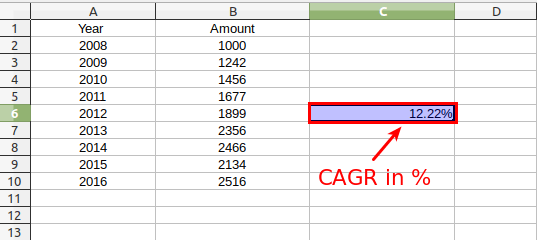# How to calculate CAGR (Compound Annual Growth Rate) in Excel

CAGR or Compound Annual Growth Rate is one the most often used financial tool to evaluate an investment over a time period.

You can use our online CAGR Calculator to easily get the CAGR value with in-depth table report and chart graphics. In the case of no internet access you can use Microsoft Excel ( Linux alternative for Excel is LibreOffice Calc or OpenOffice Calc) to calculate the CAGR.

## CAGR formula for Excel :

The formula for CAGR that you can use in Excel is:

CAGR = ( EV / SV)^(1/n)-1

where:

EV = Investment's ending value
SV = Investment's starting value
n = Time (months, years, etc.)

Let's see how to use the above formula in excel. Suppose we have following data for year and the investment value in the respective year.

 YEAR AMOUNT 2008 1000 2009 1242 2010 1456 2011 1677 2012 1899 2013 2356 2014 2466 2015 2134 2016 2516

### Step 1

You can use the above data to fill inside the Excel. Just name the column A as "YEAR" and Column B as the "AMOUNT" or value . For instance, in the image shown below, "A1" cell is assigned for "YEAR" and "B1" cell for "AMOUNT". So the "YEAR" value starts from A2 cell and ends at A10 cell. Similarly, the "AMOUNT" value starts from B2 and goes to B10. This makes the the value in the B2 cell (i.e, 1000) as the investment's starting value (SV) and value in B10 cell (i.e, 2516) as the investment's ending value (EV).### Step 2

Now, we have all values that can be applied in the above mentioned formula. In your Excel sheet, just select any cell of the "C column", in my case I selected cell "C6" ( you can choose any cell). Then, type the following as given in the image below:

=(B10/B2)^(1/8)-1

Here the value of n is 8. The invested starts in 2008, so the first year is counted as 0. From 2009 to 2016, the total number of year is 8. Hence, the value of n is 8.### Step 3

Next, hit "enter" and you will get the result inside the same cell (C6) and in the function (fx) input area. In this case, the CAGR value will be 0.122247972853454.

This result is nothing but the evaluation of the following expression : CAGR = (B10/B2)^(1/8)-1 = (2516/1000)^(1/8)-1 = 0.122247972853454### Step 4

In financial world, CAGR is commonly expressed in percentage. To get the value in percentage and rounded-off, select the "%" icon given inside the menu panel. The result in this case would be "12.22%", as shown in the image below.That's it, this is how you can calculate CAGR or compound annual growth rate for an investment using Excel.

Credit for modification suggestion: Charles Zufelt